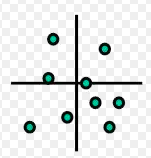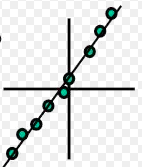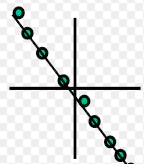+

# Correlations in Research

Author: Sophia Tutorial
##### Description:

Identify correlations in psychological research.

(more)### Developing Effective Teams

*No strings attached. This college course is 100% free and is worth 1 semester credit.

37 Sophia partners guarantee credit transfer.

299 Institutions have accepted or given pre-approval for credit transfer.

* The American Council on Education's College Credit Recommendation Service (ACE Credit®) has evaluated and recommended college credit for 32 of Sophia’s online courses. Many different colleges and universities consider ACE CREDIT recommendations in determining the applicability to their course and degree programs.

Tutorial
what's covered
This tutorial will cover the topic of correlation in psychological research. Our discussion breaks down as follows:

1. Correlation
2. Strength of Correlation
3. Direction of Correlation
4. Correlation and Causation

## 1. Correlation

You may recall the concept of a correlational study from the previous tutorial on the different forms of non-experimental research. A correlational study examines data from different sources and draws conclusions about how different psychological phenomena are related, or relate to outside factors.

EXAMPLE

For example, researchers might study stress and yoga, and yoga's affect on reducing stress. Or, another example would be a correlational study on teens and their use of social media.

Essentially, a correlation study takes different factors and tries to examine the relationship between them. This is commonly used in media, as well as in psychology itself, so it's an important concept to know.

Now, a correlation in the correlational study refers to how closely related different events, behaviors, or variables are to each other. Remember, a variable is anything that can be changed and can be measured, that can have an affect on research.

EXAMPLE

For example, variables that we might study in an experiment are the amount of sleep, age, or test scores.

terms to know
Correlational Study
A study that examines data from different sources to draw conclusions about how different psychological phenomena are related or relate to outside factors
Correlation
How closely related different events, behaviors, or variables are to each other

## 2. Strength of Correlation

There are two different factors that need to be determined when evaluating correlation: the strength of the correlation, and the direction.

First, the strength of a correlation means how strong the relationship is between the two factors. In other words, how closely related are they to each other? This can be represented in two different ways.

Mathematically, we could represent this by what is called the correlational coefficient. This is a scale from positive 1 all the way to negative 1, including all of the decimals in between. The closer you are to either plus 1 or negative 1, the stronger the relationship, or correlation, is. The closer you are to zero--in the middle--the weaker the relationship is.

Visually, we can represent the strength of a correlation by plotting out the relationships on a graph, looking to see how close the information is to forming a line of some sort.

The more scattered, or spread out, the data is, the more likely it is that there is no relationship between the variables, as shown in the graph below. This illustrates weak correlation.On the other hand, in the graph below, you can see how close the dots are to forming a line. This indicates a very strong relationship between the variables, so chances are there is correlation between the two.## 3. Direction of Correlation

Besides strength, it is also important to note the direction of the relationship between the two variables.

There can be a positive relationship, or a positive correlation, which is when one factor increases, the other one also increases, as illustrated in the graph below.EXAMPLE

If, for example, when somebody gets more sleep--when the amount of sleep goes up--their test scores also go up. In this case, there is a positive correlation between sleep and test scores.

On the other hand, there might be a negative correlation. Now, this isn't "negative" in that sense that it is bad in any way; it's simply a different kind of relationship. A negative correlation shows that when one variable goes up, the other one decreases, as illustrated in the graph below.EXAMPLE

If, for example, research shows that when somebody gets older, the amount of sleep that they need gets smaller, this would demonstrate a negative correlation between the two variables of age and sleep. Meaning, when somebody is very young, they need lots of sleep, and when they are older, they might need less sleep.

terms to know
Positive Correlation
A relationship where when one factor increases, so does the other
Negative Correlation
A relationship where when one factor increases, the other decreases

## 4. Correlation and Causation

It's important to realize, when discussing correlation, that correlation does not equal causation. Simply because you find a relationship between two different factors, doesn't mean that one causes the other one to happen. There might be an entirely different factor involved. There may be some causation going on, but not necessarily between the two correlated variables.

EXAMPLE

A study once found that when there was an increase in ice cream sales, there was also an increase in crime. Does this mean that ice cream makes people violent? No. In fact, there was a completely different factor that was affecting the results, that wasn't being considered, which is temperature. Higher temperatures made people want to eat ice cream and it also made people more violent and more apt to commit crimes.

So, you can see that just because you see a relationship, doesn't mean that there is causation. Other experimental methods, like experiments, can prove that there is actually some causation.

big idea
Correlation does not equal causation.

term to know
Causation
A relationship where one factor leads to and makes another factor occur

summary
Today we learned about correlational studies, which examine data from different sources and draws conclusions about the relationship between them. Correlation refers to how closely related these different events, behaviors, or variables are to each other. The two different factors that need to be determined when evaluating correlation are the strength of correlation and the direction of correlation. The strength of correlation refers to how strong the relationship is between two factors, and it can be represented mathematically or visually. The direction of correlation can be either positive (when one variable increases, so does the other), or negative (when one variable increases, the other decreases).

Lastly, we learned that correlation does not equal causation, which is a relationship where one factor leads to and causes another factor to occur.

Good luck!

Source: THIS WORK IS ADAPTED FROM SOPHIA AUTHOR ERICK TAGGART.

Terms to Know
Causation

A relationship where one factor leads to and makes another factor occur

Correlation

How closely related different events, behaviors, or variables are to each other

Correlational Study

A study that examines data from different sources to draw conclusions about how different psychological phenomena are related or relate to outside factors

Negative Correlation

A relationship where when one factor increases, the other decreases

Positive Correlation

A relationship where when one factor increases, so does the other

Rating